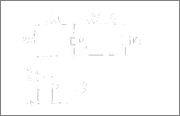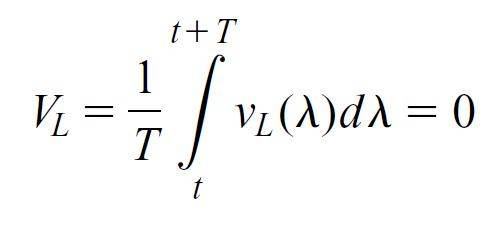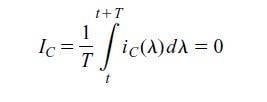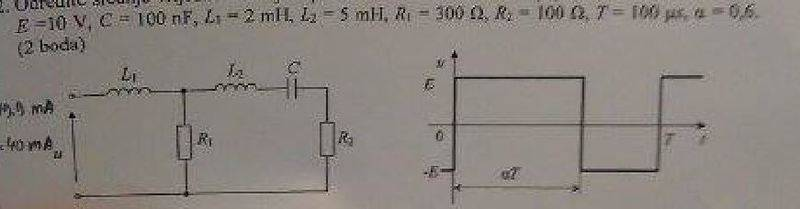# Finding average current through resistors R1 and R2

## Homework StatementFind the average currents through resistors R1 and R2

## The Attempt at a Solution

I have a question , how do I analyse this circuit besides solving differential equations using laplace transform which takes too much time, what is really confusing me is the waveform of the voltage u(t) . Well current that goes through R2 is the same current that goes through the capacitor therefore average value should be zero , Ic(0) = IR2(0) = 0 A, also we know that average voltages are zero on the inductor UL(0) = 0 , so whole U(0) = UR1(0) , so IR1(0) 0 UR1(0) / R1 does this make sense?

( currents and voltages with zero in brackets represent avg values)

Last edited:

Hesch
Gold Member
current that goes through R2 is the same current that goes through the capacitor therefore average value should be zero
I think that the average currents of the absolute values of the currents are meant here?
I assume that your lower figure is to be continued as a square wave?

I think that the average currents of the absolute values of the currents are meant here?
I assume that your lower figure is to be continued as a square wave?
Yes it's periodically square wave , not sure whether it asks to find absolute values, it says find average value of the currents, therefore avg value can be negative , if that is what you meant.

Hesch
Gold Member
Yes it's periodically square wave , not sure whether it asks to find absolute values
If u(t) is a symmetrical square wave then

IR1,avg = 1/T 0TIR1 dt → 0 for T → ∞

and so will IR2,avg

but 1/T 0Tabs(IR1) dt will not.

The exercise doesn't make sense, if you are not using absolute values.

gneill
Mentor
Every now and then I come across an author who uses "average" when they really mean RMS when dealing with AC circuits. Is it possible that this is the case here?

Are there component values given, or are you to find a result symbolically only?

Have you considered expressing the input signal as a Fourier series? The L's and C in combination with their associated resistors are going to limit the range of terms that can contribute significantly to the currents.

Hesch
Gold Member
an author who uses "average" when they really mean RMS
Well, often (absolute) average currents are calculated in rectifiers/power supplies.

Average currents and voltages are very often calculated in power electronics, for devices like DC-DC converters and rectifiers.

The average inductor voltage is zero:screenshot software
If this wasn't the case every period you would have more Vs in the inductor.
The average capacitor current is zero:
http://postimage.org/][/PLAIN] [Broken]
photo host

If this wasn't the case every period you would have more As in the capacitor.

Last edited by a moderator:
Hesch
Gold Member
#7: Yes, you could calculate that, but since power electronics have a lot to do with energy, it's more interesting to calculate the average IL and VC because

EL = ½*IL2* L
and
EC = ½*VC2*C

In power electronics capacitors and inductors are there to store energy ( temporary).

•Ivan Antunovic
NascentOxygen
Staff Emeritus
Yes it's periodically square wave
This would be a good question for a multi-choice test were the input specified as rectangular with duty-cycle 50%.•Ivan Antunovic
This would be a good question for a multi-choice test were the input specified as rectangular with duty-cycle 50%.This was actually a first task in the exam this week from the power electronics course. I just simplified the task not bothering you with with numbers.screengrab
As you can see from the picture it is a rectangular wave signal with duty-cycle ≠ 50%.
(notice different notation for duty cycle 'α')
α = D = duty cycle = 0.6

•NascentOxygen
NascentOxygen
Staff Emeritus
This was actually a first task in the exam this week from the power electronics course.
Now I'm confused. Are you clarifying the context of your circuit in post #1?
As you can see from the picture it is a rectangular wave signal with duty-cycle ≠ 50%.
(notice different notation for duty cycle 'α')
α = D = duty cycle = 0.6
So you now realise how to solve for average resistor currents in your circuit in post #1? The examiner almost certainly does, in this case, intend average and nothing else.

Now I'm confused. Are you clarifying the context of your circuit in post #1?

So you now realise how to solve for average resistor currents in your circuit in post #1? The examiner almost certainly does, in this case, intend average and nothing else.
Yes picture is clarification from the context of the circuit in post #1. I figured out how to solve this circuit in my 1st post , just wasn't sure if it was correct way , needed your approval. Asked a friend of mine , he agreed with my solution
Ic(0) = IR1(0) = 0 and U(0) = UR1(0) -----> IR1(0) = UR1(0) / R1
if you put numbers in:
U(0) = 10 * 0.6 - 10 * (1-0.6 ) = 2 V
IR1(0) = UR1(0) / R1 = 2 / 300 = 6.67 mA